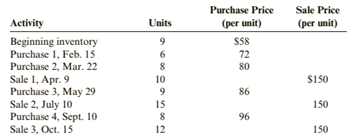Chapter 6, Problem 67BPSB### Cornerstones of Financial Accounti...

4th Edition
Jay Rich + 1 other
ISBN: 9781337690881

#### Solutions

Chapter
Section### Cornerstones of Financial Accounti...

4th Edition
Jay Rich + 1 other
ISBN: 9781337690881
Textbook Problem
111 views

# Inventory Costing MethodsEin Company began operations in February 2019. Ein’s accounting records provide the following data for the remainder of 2019 for one of the items the company sells:Ein uses a perpetual inventory system. All purchases and sales were for cash.Required:1. Compute cost of goods sold and the cost of ending inventory using FIFO.2. Compute cost of goods sold and the cost of ending inventory using LIFO.3. Compute cost of goods sold and the cost of ending inventory using the average cost method. ( Note: Use four decimal places for per-unit calculations and round all other numbers to the nearest penny.)4. Prepare the journal entries to record these transactions assuming Ein chooses to use the FIFO method.5. CONCEPTUAL CONNECTION Which method would result in the lowest amount paid for taxes?

To determine

(a)

Inventory costing methods:

FIFO, LIFO and average cost method, are those method which used for calculation of closing inventory and cost of goods sold.

The cost of ending inventory and the cost of goods sold using the FIFO.

Explanation

The given information is as follows:

Total available units are:

Opening inventory=9 units @ $58 each Purchases=6 units @$72 eachPurchases=8 units @ $80 eachPurchases=9 units @$86 eachPurchases=8 units @ \$96 each

Total Purchased units = 31

Total available quantity = 31+9= 40 units

Sales=37 units

Closing inventory =Beginning inventory + PurchaseSales=9+3137=3 units

Calculation of Closing Inventory as per FIFO Method:

Under this method, which material purchased first, issued first for production

To determine

(b)

Inventory costing methods:

FIFO, LIFO and average cost method, are those method which used for calculation of closing inventory and cost of goods sold.

The cost of ending inventory and the cost of goods sold using the LIFO.

To determine

(c)

Inventory costing methods:

FIFO, LIFO and average cost method, are those method which used for calculation of closing inventory and cost of goods sold.

The cost of ending inventory and the cost of goods sold using the average cost method.

To determine

(d)

Inventory costing methods:

FIFO, LIFO and average cost method, are those method which used for calculation of closing inventory and cost of goods sold.

The journal entries for recording the transaction on the basis of FIFO method.

To determine

(e)

Inventory costing methods:

FIFO, LIFO and average cost method, are those method which used for calculation of closing inventory and cost of goods sold.

The method for determining the lowest amount paid for tax.

### Still sussing out bartleby?

Check out a sample textbook solution.

See a sample solution

#### The Solution to Your Study Problems

Bartleby provides explanations to thousands of textbook problems written by our experts, many with advanced degrees!

Get Started

#### Find more solutions based on key concepts